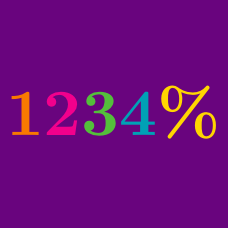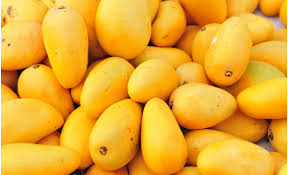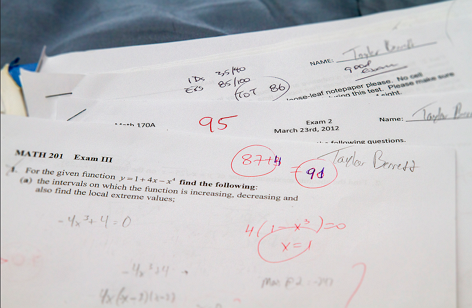Basic Mathematics

# Percentages: Level 2 ChallengesIn a box of mangoes, 80% of them are high-quality while 20% are low-quality. If 10 low-quality mangoes are removed, then 96% of the rest will be high-quality.

What is the total number of mangoes from the start?$\LARGE\color{#20A900}{\sqrt{100\%}=\ ?}$

There are 100 people in a room, exactly 99% are physicist. How many physicist must leave the room to bring down the percentage of physicist to exactly 98%?

If $x=80\%$, then by what percent is $x$ larger than $x^2$?

There are 40 students in the classroom.

• 50% of students wear both glasses and sweaters.
• Among the students who don't wear glasses, 75% of them wear sweaters.

If two students wearing both glasses and sweaters leave the classroom, then which of the following is a possiblity for the percentage of students who wear neither glasses nor sweaters?

×

Problem Loading...

Note Loading...

Set Loading...